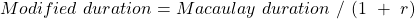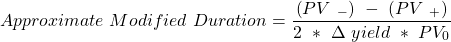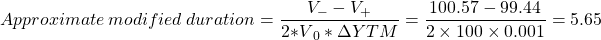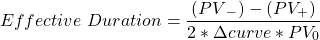Concept 85: Macaulay, Modified, and Effective Ddurations | IFT World
101 Concepts for the Level I Exam

# Concept 85: Macaulay, Modified, and Effective DdurationsBond duration measures the sensitivity of the bond’s price to changes in interest rates. The three common measures of duration are:

Macaulay duration: The weighted average of the time to receipt of coupon interest and principal payments.

Modified duration: A linear estimate of the percentage price change in a bond for a 100 basis points change in its yield-to-maturity.A 12% annual-pay bond has 10 years to maturity. The bond is currently trading at par. Assuming a 10 basis-points change in yield-to-maturity, calculate the bond’s approximate modified duration.

Solution:

The bond is priced at par which means that the initial YTM = coupon rate = 12% and V0 = 100.

ΔYTM = 0.001

V = 100.57

N = 10, PMT = 12, FV = 100, I/Y = 11.9; CPT à PV = 100.57

V+ = 99.44

I/Y = 12.1; CPTà PV = 99.44Effective duration: The linear estimate of the percentage change in a bond’s price that would result from a 100 basis points change in the benchmark yield curve.